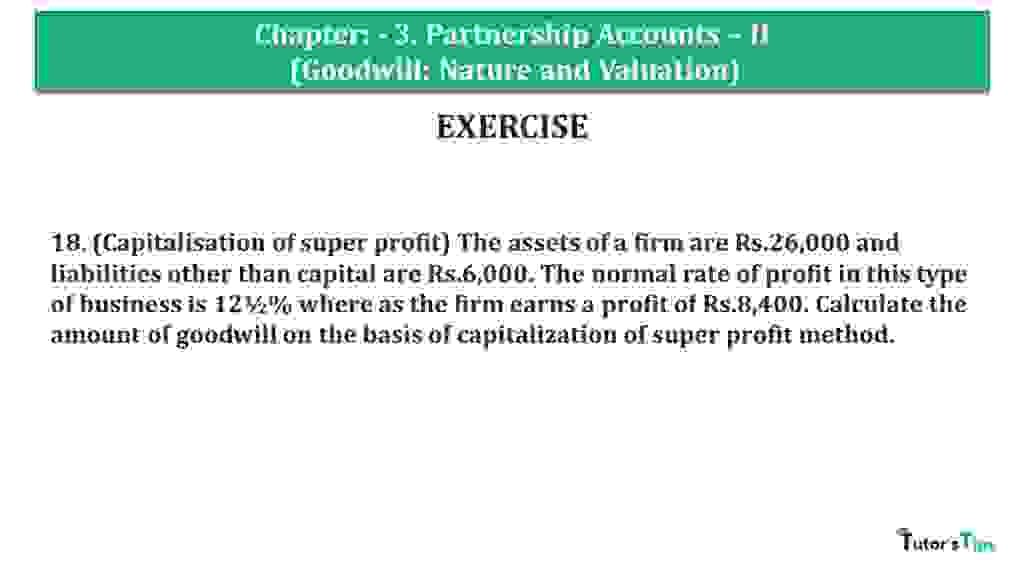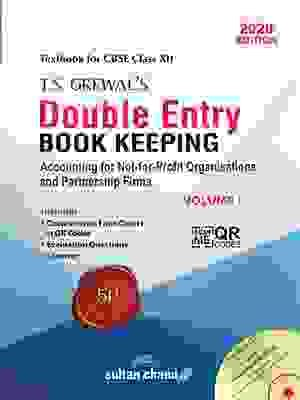# Question 18 Chapter 3 of +2 Part-1 – USHA Publication 12 Class Part – 1Question 18 Chapter 3 of +2-Part-1

18. (Capitalisation of super profit) The assets of a firm are Rs.26,000 and liabilities other than capital are Rs.6,000. The normal rate of profit in this type of business is 12½% where as the firm earns a profit of Rs.8,400. Calculate the amount of goodwill on the basis of capitalization of super profit method.

### The solution of Question 18 Chapter 3 of +2 Part-1: –

 Normal Profit = Capital Employed X Normal Rate of Return 100 = 20,000 (i.e. 26,000-6,000) X 10 100 = 2,500

 Super Profit = Actual Profit – Normal Profit = 8,400 – 2,500 = 5,900

 Goodwill = Super Profit X 100 Normal Rate of Return = 5,900 X 100 12.5 = 47,200

Thanks, Please Like and share with your friends

Comment if you have any questions.

Also, Check out the solved question of previous Chapters: –

## Usha Publication – Accountancy PSEB (Class 12) – Volume II – SolutionVol. I: Accounting for Not-for-Profit Organizations and Partnership Firms